GFG App
Open AppBrowser
Continue

# R – Merge Multiple DataFrames in List

In this article, we will discuss how to merge multiple data frames in the list using R programming language.

## Method 1: Using merge()

First create more than two data frames, so that we could able to merge them. Now, use merge() function along with the required parameters.

Syntax :

merge( x=dataframe , y=dataframe , by= primary_key_of_the_dataframe )

Now, pass this merged dataframe to as.list() to convert it into a list.

Syntax:

as.list(merged_dataframe)

Example 1: Merge multiple dataframes in a list

## R

 `df = ``data.frame``( ` `  ``id=``c``(1, 2, 3),  ` `  ``name=``c``(``"karthik"``, ``"chandu"``, ``"nandu"``)) ` ` `  `df1 = ``data.frame``( ` `  ``id=``c``(1, 2, 3),  ` `  ``branch=``c``(``"IT"``, ``"CSE"``, ``"CSE"``)) ` ` `  `merg = ``merge``(x=df, y=df1, by=``"id"``) ` `print``(``as.list``(merg)) `

Output :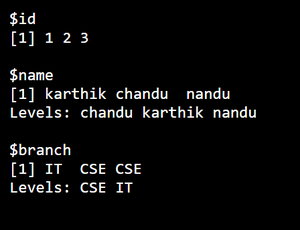Example 2: Merge multiple dataframes in list

## R

 `df = ``data.frame``( ` `  ``id=``c``(1, 2, 3),  ` `  ``name=``c``(``"karthik"``, ``"chandu"``, ``"nandu"``)) ` ` `  `df1 = ``data.frame``( ` `  ``id=``c``(1, 2, 3),  ` `  ``branch=``c``(``"IT"``, ``"CSE"``, ``"CSE"``)) ` ` `  `df2 = ``data.frame``( ` `  ``id=``c``(1, 2, 3),  ` `  ``company=``c``(``"TCS"``, ``"Accenture"``, ``"Infosys"``)) ` ` `  `merg = ``merge``(x=df, y=df1, z=df2, by=``"id"``) ` `print``(``as.list``(merg)) `

Output :## Method 2 : Using cbind() function

If we want to merge more than two dataframes we can use cbind() function and pass the resultant cbind() variable into as.list() function to convert it into list .

Syntax :

cbind( df1 , df2 . df3 , . . . dfn )

Example 1: Merge multiple dataframes in list

## R

 `df = ``data.frame``(name=``c``(``"karthik"``, ``"chandu"``, ``"nandu"``)) ` `df1 = ``data.frame``(branch=``c``(``"IT"``, ``"CSE"``, ``"CSE"``)) ` `df2 = ``data.frame``(company=``c``(``"TCS"``, ``"Accenture"``, ``"Infosys"``)) ` ` `  `merg = ``cbind``(df, df1, df2) ` `print``(``as.list``(merg)) `

Output :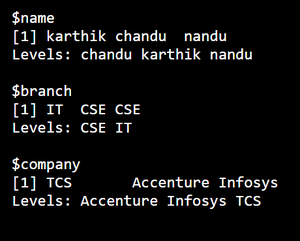Example 2: Merge multiple dataframes in list

## R

 `df = ``data.frame``(name=``c``(``"karthik"``, ``"chandu"``, ``"nandu"``)) ` `df1 = ``data.frame``(collage_name=``c``(``"VFSTR"``, ``"VMTW"``, ``"IIT"``)) ` `df2 = ``data.frame``(place=``c``(``"Guntur"``, ``"Hyderabad"``, ``"Kharagpur"``)) ` `df3 = ``data.frame``(proper=``c``(``"yellandu"``, ``"yellandu"``, ``"yellandu"``)) ` ` `  `merg = ``cbind``(df, df1, df2, df3) ` `print``(``as.list``(merg)) `

Output :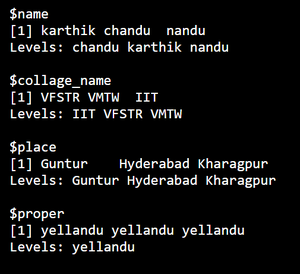## Method 3 : Using tidyverse

If we want to merge more than two dataframes we can use tidyverse library too. Here a first an inner join is created for all the participating dataframes and then that is converted to a list as above.

Syntax:

reduce(inner_join, by=”common column”)

Example 1: Merge multiple dataframes in list

## R

 `library``(``"tidyverse"``) ` ` `  `df1 = ``data.frame``( ` `  ``id=``c``(1, 2, 3),  ` `  ``name=``c``(``"karthik"``, ``"chandu"``, ``"nandu"``)) ` ` `  `df2 = ``data.frame``( ` `  ``id=``c``(1, 2, 3), ` `  ``Gender=``c``(``"Male"``, ``"Female"``, ``"Male"``)) ` ` `  `df3 = ``data.frame``( ` `  ``id=``c``(1, 2, 3),  ` `  ``address=``c``(``"Yellandu"``, ``"Yellandu"``, ``"Yellandu"``)) ` ` `  `data = ``list``(df1, df2, df3) ` `as.list``(data % > % ``reduce``(inner_join, by=``"id"``)) `

Output :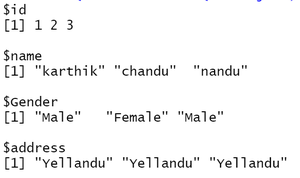Example 2 : Merge multiple dataframes in list

## R

 `library``(``"tidyverse"``) ` ` `  `df1 = ``data.frame``( ` `  ``id=``c``(1, 2, 3), ` `  ``name=``c``(``"karthik"``, ``"chandu"``, ``"nandu"``)) ` ` `  `df2 = ``data.frame``( ` `  ``id=``c``(1, 2, 3), ` `  ``Gender=``c``(``"Male"``, ``"Female"``, ``"Male"``)) ` ` `  `df3 = ``data.frame``( ` `  ``id=``c``(1, 2, 3),  ` `  ``address=``c``(``"Yellandu"``, ``"Yellandu"``, ``"Yellandu"``)) ` ` `  `df4 = ``data.frame``( ` `  ``id=``c``(1, 2, 3),  ` `  ``father_name=``c``(``"Ramana"``, ``"Radha"``, ``"krishna"``)) ` ` `  `data = ``list``(df1, df2, df3, df4) ` `as.list``(data % > % ``reduce``(inner_join, by=``"id"``)) `

Output :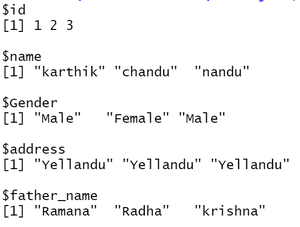My Personal Notes arrow_drop_up